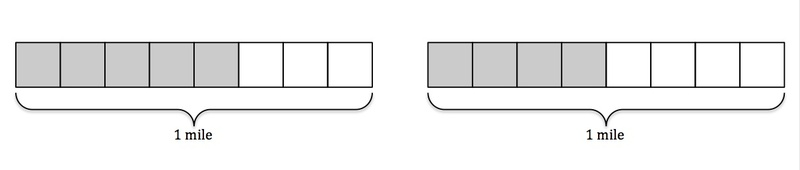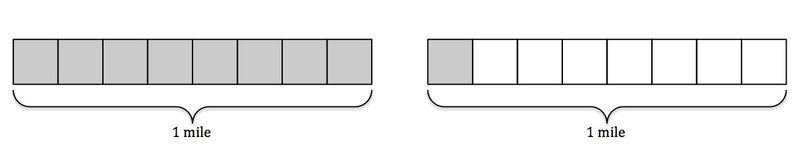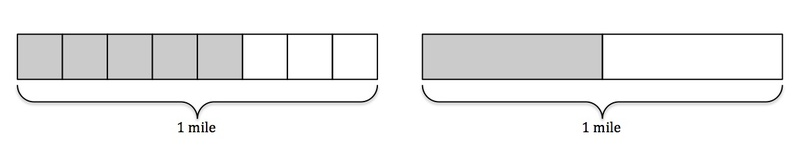# Jog-A-Thon

Alignments to Content Standards: 5.NF.A.1

Alex is training for his school's Jog-A-Thon and needs to run at least $1$ mile per day. If Alex runs to his grandma's house, which is $\frac58$ of a mile away, and then to his friend Justin's house, which is $\frac12$ of a mile away, will he have trained enough for the day?

## IM Commentary

The purpose of this task is to present students with a situation where it is natural to add fraction with unlike denominators; it can be used for either assessment or instructional purposes. Teachers should anticipate two types of solutions: one where students calculate the distance Alex ran to determine an answer, and one where students compare the two parts of his run to benchmark fractions.

Actually calculating how far Alex ran illustrates an understanding of the process of adding fractions with unlike denominators. If students take this approach, the teacher should prompt students to show work that clearly indicates the thought process. If students approach the problem by comparing the given fractions to benchmark fractions, the teacher should expect to see less written work. This method requires fraction number sense. Both methods are perfectly reasonable ways to solve this problem, and students should be aware of both methods if this task is used in an instructional setting.

## Solutions

Solution: Calculating

First, let's determine how far Alex has run. He ran $\frac58$ of a mile to his grandma’s house, and then $\frac12$ of a mile to Justin’s house. Together, Alex ran $\frac58$ + $\frac12$ miles.

To add fractions, we must have a common denominator for $\frac58$ and $\frac12$. A common denominator of $\frac58$ and $\frac12$ is $8$, so we can use that.

$$\frac12 = \frac{1\times 4}{2\times 4} = \frac48$$

Now that we have a common denominator we can calculate: $$\frac58 + \frac12 = \frac58 + \frac48 = \frac98 = 1 \frac18.$$

In total, Alex ran $1\frac18$ miles. (See Accompanying Images)

Since Alex’s minimum training distance is $1$ mile and he trained more than $1$ mile, the answer is yes; Alex did train enough for the day.

• Accompanying Images:

Alex ran $\frac58$ of a mile to his grandma's house and $\frac12$=$\frac48$ of a mile to his friend Justin's house. The picture on the left represents Alex's run to his grandma's house, and the picture on the right represents Alex's run to his friend Justin's house.By combining the two trips,we can see that Alex ran $1$ full mile and an additional $\frac18$ of a mile, or $1\frac18$ miles.

Solution: Estimating

To determine whether or not Alex has trained enough for the day we can compare the distance he ran with his minimum training distance. We don't need to calculate the exact distance Alex ran but only whether Alex ran more or less than $1$ mile.

Alex ran $\frac58$ of a mile to his grandma’s house, and we know that

$$\frac58\gt\frac48=\frac12$$

So Alex ran more than half of a mile to his grandma’s house.

He then ran $\frac12$ of a mile to his friend Justin’s house. Since Alex ran more than half of a mile and then an additional half of a mile, we know that he ran more than a full mile. (See Accompanying Images)

Because Alex ran more than his minimum training distance of $1$ mile the answer is yes; Alex has trained enough for the day.

• Accompanying Images:

First we will start with a picture representing $1$ mile.Now we have pictures that represent the portions of a mile that Alex ran on each of his two tips. The picture on the left represents the $\frac58$ of a mile that Alex ran to his grandma's house. The picture on the right represents the $\frac12$ of a mile that Alex ran to his friend Justin's house.Stacking these images on top of each other, as shown below, makes it easy to see that $\frac58$ > $\frac12$.By combing the shaded portions, representing the distance Alex actually ran, and comparing this to our original model of $1$ mile,we can clearly see that Alex ran more than $1$ mile.